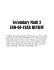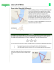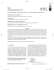# Practice - buskemath

## Transcription

Practice - buskemath
```Name
Class
14-3
Date
Practice
Form G
Right Triangles and Trigonometric Ratios
Find the values of the six trigonometric functions for the angle in standard
position determined by each point.
1. (3, 4)
2. (12, 5)
3. (2, 1)
4. ( 5 , 2)
5. A hiker is standing on one bank of a river. A tree stands on the opposite bank,
which is 750 ft away. A line from the top of the tree to the ground at the hiker’s
feet makes an angle of 12° with the ground. How tall is the tree?
6. In ΔABC, find each value as a fraction and as a decimal. Round to the
nearest hundredth.
a. cos A
b. csc A
c. tan B
d. sec B
e. cot A
f. csc B
2
. Draw a diagram and find
3
each value in fraction form and in decimal form. Round your answer to the
nearest tenth, if necessary.
7. In ΔABC, C is a right angle and tan A 
a. cos A
b. tan B
c. sin A
d. cot B
e. sec A
f. csc B
Find each length x. Round to the nearest tenth.
8.
9.
Prentice Hall Gold Algebra 2 • Teaching Resources
23
Name
Class
14-3
Date
Practice (continued)
Form G
Right Triangles and Trigonometric Ratios
10. A kite string makes a 62° angle with the horizontal, and 300 ft of string is let
out. The string is held 6 ft of the ground. How high is the kite?
In ΔDEF, D is a right angle. Find the remaining sides and angles. Round answers
to the nearest tenth.
11. f = 8, e = 15
12. f = 1, e = 2
13. f = 2, e = 1
14. f = 1, d = 500
15. d = 21, e = 8
16. e = 5, f = 1
17. You are designing several access ramps. What angle would each ramp make with the
ground, to the nearest 0.1°?
a. 20 ft long, rises 16 in.
b. 8 ft long, rises 8 in.
c. 12 ft long, rises 6 in.
d. 30 ft long, rises 32 in.
e. 4 ft long, rises 6 in.
f. 6 ft long, rises 14 in.
Sketch a right triangle with  as the measure of one acute angle. Find the other
five trigonometric ratios of .
18. cos 
4
11
19. cos  
7
12
20. cos 
14
6
21. cos 
9
16
22. sin  = 0.45
23. sec  = 7.6
1
, describe a method you could use to find all the
2
angles between 0° and 360° that satisfy this equation.
24. Open-Ended If cos 
25. Reasoning Show that if C is a right angle in ΔABC, then the area of ΔABC
is
bc
sin A.
2
Prentice Hall Gold Algebra 2 • Teaching Resources
24
```

### AS Entrance Examination Sample Paper Mathematics Instructions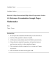### pdf file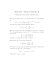### installation of an angle board * * * thermopian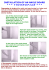### Test 2 Review 1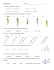### Problem Solving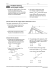### Review12.1-12.6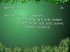### Higher Relationships and Calculus NAB Revision Pack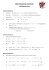### Inverse Trig Functions You know how to find the missing side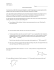### Test 2 Review 2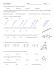### Document 6588179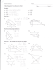### Torque/Cross Product Worksheet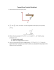### Infinite Algebra 2